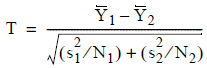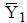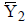# 5.4.5 - Two Sample T-Test for Equal Means - Teradata Warehouse Miner

## Teradata Warehouse Miner User Guide - Volume 3Analytic Functions

Product
Release Number
5.4.5
Published
February 2018
Language
English (United States)
Last Update
2018-05-04
dita:mapPath
yuy1504291362546.ditamap
dita:ditavalPath
ft:empty

For the paired t test, a one-to-one correspondence must exist between values in both samples.

The test is whether paired values have mean differences which are not significantly different from zero. It assumes differences are identically distributed normal random variables, and that they are independent.

The unpaired t test is similar, but there is no correspondence between values of the samples.

It assumes that, within each sample, values are identically distributed normal random variables, and that the two samples are independent of each other. The two sample sizes may be equal or unequal. Variances of both samples may be assumed to be equal (homoscedastic) or unequal (heteroscedastic). In both cases, the null hypothesis is that the population means are equal. Test output is a p-value which compared to the threshold determines whether the null hypothesis should be rejected.

Two methods of data selection are available for the unpaired t test:
• The first, the “T Unpaired”. simply selects the columns with the two unpaired datasets, some of which may be NULL.
• The second, “T Unpaired with Indicator”, selects the column of interest and a second indicator column which determines to which group the first variable belongs.
If the indicator variable is negative or zero, it will be assigned to the first group; if it is positive, it will be assigned to the second group.

The two sample t tests for unpaired data are defined as shown below (though calculated differently in the SQL):

 H0: μ1 = μ2 Ha: μ1 ≠ μ2 Test Statistic:where N 1 and N 2 are the sample sizes,andare the sample means, and s 1 2 and s 2 2 are the sample variances.